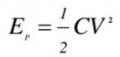# Exponential decay graph

Joined Feb 10, 2016
68
how to draw waveform when the switch is closed for lone time the capacitor is behaving like an open source so 10 volts at the node and when the switch moves to 2nd position v(0)=10e^-t/tau t=Rc = 10k x 100uf
V(0)=10e^-t
so i plotted the graph..is this alright because voltage and current decrease exponentially.
And energy stored is w=1/2Cv^2(1-e^(-2t/tao))
So putting t=0

w=1/2(100uf)(10)^2(1-1)
w=0

#### RBR1317

Joined Nov 13, 2010
712
Whenever there is a switching transient in a resistive circuit with a single capacitor or inductor, there will be a first-order transient response as shown in the attached diagram (voltage is shown but it would be the same for current). Any first-order transient response is characterized by the following:

1) Is the transient an exponential rise or an exponential decay?

2) The magnitude of the transient, V_T.

3) The capacitor or inductor time constant.

4) The zero level, i.e. some circuits may have a constant offset from 0 and the transient response must be offset by the same amount.

If you can answer these four questions correctly, then you are guaranteed to have the correct first-order transient response.

#### Attachments

Joined Feb 10, 2016
68
When its at switch 2 its an exponential decay but if its connected to another source then my formula would be for exponential rise
v(t)(1-e^(-t/tau)) ?

#### anhnha

Joined Apr 19, 2012
905
When its at switch 2 its an exponential decay but if its connected to another source then my formula would be for exponential rise
v(t)(1-e^(-t/tau)) ?
No, your initial formula is correct Vo =10e^-t.
And energy stored is w=1/2Cv^2(1-e^(-2t/tao))
This is not correct. What you calculated here how much energy is dissipated not what stored.
Energy stored in a capacitor:Where V is the voltage across the capacitor.

Last edited:

#### RBR1317

Joined Nov 13, 2010
712
When its at switch 2 its an exponential decay but if its connected to another source then my formula would be for exponential rise
v(t)(1-e^(-t/tau)) ?
Correct. Switch position 2 causes an exponential decay transient. If switched back to position 1 the transient would be an exponential rise in the voltage across the capacitor. It gets more interesting if it is switched back to position 1 before the capacitor has been fully discharged.